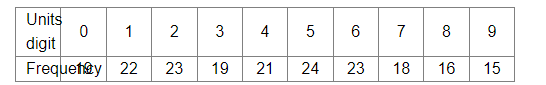# On one page of a telephone directory, there are 200 phone numbers.Question:

On one page of a telephone directory, there are 200 phone numbers. The frequency distribution of their units digits is given below:One of the numbers is chosen at random from the page. What is the probability that the units digit of the chosen number is
(i) 5?
(ii) 8?

Solution:

Total phone numbers on the directory page  = 200

(i) Number of numbers with units digit 5 = 24

Let E1 be the event that the units digit of selected number is 5.

$\therefore$ Required probability $=P\left(E_{1}\right)=\frac{24}{200}=0.12$

(ii) Number of numbers with units digit 8 = 16

Let E2 be the event that the units digit of selected number is 8.

$\therefore$ Required probability $=P\left(E_{2}\right)=\frac{16}{200}=0.08$